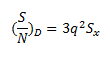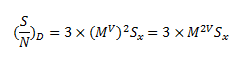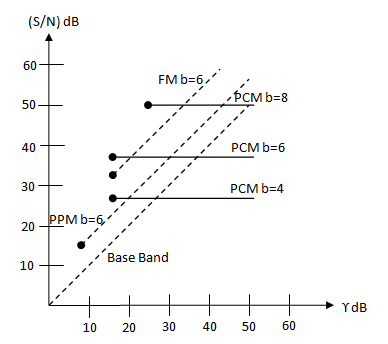# Comparison of PCM and Analog Modulation

## Compairing PCM and Analog Modulation

In this tutorial we shall compare PCM and Analog Modulation in detail.

The threshold effect in PCM is similar to a property of analog modulation methods such as FM or PPM.

The property is that , these systems tend to reduce the wideband noise above the threshold levels.

The PCM also provides the wideband noise reduction if it is operated above its threshold which is given by:Where q=2for binary PCM and q= Mfor M-ary PCM.

We assume that the sampling frequency is close to the Nyquist rate and bandwidth BW = Nfm  Hz.

Then, q=Mv= Mb  where b = BW/ fis known as the bandwidth ratio.

Therefore, we have,Here,  (S/N)D = Signal to noise ratio at the destination

Sx  = Signal power at the destination

Here, it may be noted that the signal to noise ratio (S/N)D  is proportional to M2b which is much higher than the (S/N)of the wideband FM which is proportional to only b or b2.

Hence, PCM performs better than FM .

Fig.1 shows the performance of various modulation types as a function of γ .Comparison of PCM and analog communication

Fig.1

All the curves in fig.1 have been plotted for Sx= 1/2. The dots indicate the threshold points. The PCM curves have been drawn for M = 2 and v = b.

### Conclusions

Some of the important observations from fig.1 may be listed as under:

1. For PCM if b is constant , then increase in γ beyond the threshold value  γth (corresponding to the threshold point) does not increase (S/N)D  at all . Let us observe the flat PCM curves in fig.1. hence, PCM must be operated just above the threshold.
2. Near threshold, the PCM does offer some advantages over FM and PPM, with thesame values of b and (S/N)D .
3. However, this advantage is gained at the expense of more complicated and expensive circuitry.
4. The (S/N)for FM and PPM increases linearly with increase in the value of γ and becomes better than that of PCM for higher values of γ .

### Benefits of PCM

Fig.1 reveals the following benefits of using the PCM :

1. PCM allows the use of regenerative repeaters. This improves its noise performance.
2. PCM allows the transmission of analog signals in the form of digital signals.